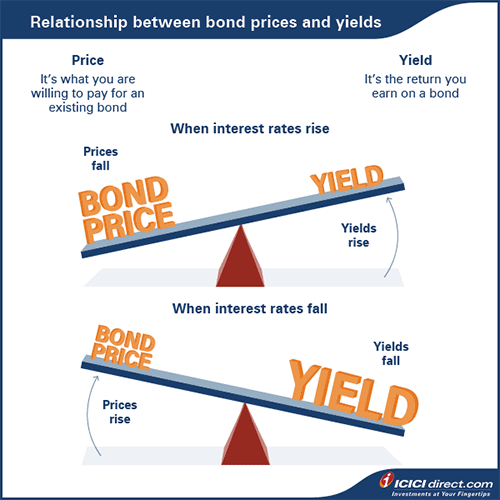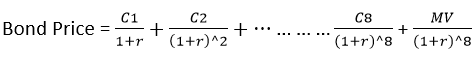Debt mutual funds have become quite popular amongst investors. As you now know, debt mutual funds are funds that invest in different fixed-income securities like bonds, G-Secs, debentures, etc. to generate returns for investors. Let’s get down to understanding how they work.

## How Debt Mutual Funds Generate Returns

Remember how we discussed in the previous chapter that debt instruments like bonds work a little like loans? A bond or debenture issuer is the borrower, and the investor (you in this case) is the lender. The interest paid on fixed income securities for the money you have “lent” is the return.

So, how do debt mutual funds work? Simplistically, there are two ways in which debt mutual funds make returns for investors:

1. Via the interest they receive
2. Through capital gains, i.e. price appreciation of bond or fixed-income security

In the first scenario, debt mutual funds that invest in multiple debt securities earn interest, which then adds on to the fund’s assets. The yield or return that you as a mutual fund investor receive is based on the interest earned from multiple investments.

On the other hand, just like equity shares are traded on stock markets, there are also debt markets where different types of debt instruments are traded.

Do you remember?

Face value of a fixed income security, like a bond, is the amount of money promised to an investor on maturity while price is the current market value of the instrument.

Here, the prices may fall or rise, just like in stock markets. If a debt mutual fund buys a security and its price rises, then they make more money over and above the interest. This, in turn, will be will be added to the net assets and increase the NAV for you as an investor. On the other hand, if the price in the market falls, then it can lower your NAV.

A bond’s price in the secondary market depends upon prevailing yield in the bond market of other similar bonds. And if you remember, a bond’s price is inversely related to yield. This means that the price of a bond will rise when yield falls and vice versa.

Do you remember?

A bond’s coupon remains fixed even through fluctuations in the market?And how does this happen, you may wonder.

If new bonds are issued at a coupon lower than old bonds, then the older bonds become more valuable.

For instance, let’s assume the government has issued a 10-year G-Sec at 7%. Then, changes in the economy and other factors cause interest rates to fall. Subsequently, the government issues a new 10-year G-Sec at 6.5%. Now, the old bond becomes more valuable because of the higher interest it pays.

Now, investors who have the 7% G-Sec can charge a “premium” in the secondary market, leading to an increase in the price of the bond. If a mutual fund holding the old bond decides to sell, then it would make more gains apart from the interest as well.

 Did you know? Fixed income securities issued by the Government of India forms the largest component of India’s debt markets.

## Breaking Down Pricing

Let’s get to the numbers to get a clearer understanding of bond pricing.

For example, say the Government of India issues a bond for 5 years with a 7% p.a. coupon paid semi-annually for a face value of Rs. 1,000. What this means is that an investor will pay Rs. 1,000 to buy the bond. The interest will be calculated as follows:

Annual Interest = Face value x Coupon rate
= Rs. 1,000 x 0.07 = Rs. 70

Since it is a semi-annual bond, you will get Rs. 35 every six months. The principal of Rs. 1,000 will be paid after 5 years. This is in case the bond is held till maturity.

However, debt mutual funds aim to make money by trading such bonds. Now let’s assume a debt mutual fund who bought these bonds wants to assess its performance in a year. What would happen then?

Here is where market dynamics and the calculation of present value of discounted future cash flows would come into play. There are three ways this could play out:

1. The bond could be trading at par, or at the same rate
2. The bond could be trading at a discount

Scenario 1: Let’s assume the interest rate doesn’t change and remains 7%

The price of the bond after a year can be calculated by discounting the remaining coupon amount and maturity value. After a year, 8 semiannual coupon payment are left.Here, C1 represents the first coupon amount i.e. Rs. 35.

r = semiannual discount rate. In this case, it is 7%/2 = 3.5%

MV = Maturity value i.e. Rs. 1,000, in this case.

Here is the present value of future discounted cash flows:

 S. No Coupon amount Semi-annual discount rate Discount period Present Value (PV) C1 35 0.035 1 35/1.035 = 33.82 C2 35 0.035 2 35/(1.035)^2 = 32.67 C3 35 0.035 3 35/(1.035)^3 = 31.57 C4 35 0.035 4 35/(1.035)^4 = 30.50 C5 35 0.035 5 35/(1.035)^5 = 29.47 C6 35 0.035 6 35/(1.035)^6 = 28.47 C7 35 0.035 7 35/(1.035)^7 = 27.51 C8 35 0.035 8 35/(1.035)^8 = 26.58 MV 1000 0.035 8 1000/(1.035)^8 = 759.41 Total 1000

We can see that sum of the present value of the future cash flow is Rs. 1,000 i.e. bond price remains unchanged.

Scenario 2: When interest rate falls i.e. becomes 6%

Using the same formula, we get the following present value:

 S No Coupon Amount Semi-annual Discount Rate Discount Period PV C1 35 0.03 1 35/1.03 = 33.98 C2 35 0.03 2 35/(1.03)^2 = 32.99 C3 35 0.03 3 35/(1.03)^3 = 32.03 C4 35 0.03 4 35/(1.03)^4 = 31.10 C5 35 0.03 5 35/(1.03)^5 = 30.19 C6 35 0.03 6 35/(1.03)^6 = 29.31 C7 35 0.03 7 35/(1.03)^7 = 28.46 C8 35 0.03 8 35/(1.03)^8 = 27.63 MV 1000 0.03 8 1000/(1.03)^8 = 789.41 Total 1035.10

If the fund sells the bond, it will make a capital gain. This would be a realized gain. But if you hold on to the bond and the asset price increases, it would be recorded as an unrealized gain. Both realized and unrealized gains would be included in the NAV and would be passed on to you.

Scenario 3: When interest rate rises and goes up to 8%

We have calculated the PV of all coupons in the following table:

 S. No Coupon Amount Semi-annual Discount Rate Discount Period PV C1 35 0.04 1 35/1.04 = 33.65 C2 35 0.04 2 35/(1.04)^2 = 32.36 C3 35 0.04 3 35/(1.04)^3 = 31.11 C4 35 0.04 4 35/(1.04)^4 = 29.92 C5 35 0.04 5 35/(1.04)^5 = 28.77 C6 35 0.04 6 35/(1.04)^6 = 27.66 C7 35 0.04 7 35/(1.04)^7 = 26.60 C8 35 0.04 8 35/(1.04)^8 = 25.57 MV 1000 0.04 8 1000/(1.04)^8 = 730.69 Total 966.34

We can see that sum of the present value of future cash flow is Rs. 966.34 i.e. the bond trades at a discount and the fund will incur a loss. It will have a negative impact on the NAV.

So, debt mutual funds use bond prices to partly determine whether to sell a fixed income security to earn capital gains or to hold it till maturity. They make these decisions for all the securities that they hold.

##### Summary
• Debt mutual funds invest in different fixed income securities to generate money for investors.
• There are two ways in which debt mutual funds make returns for investors: via interest and capital gains if they sell securities at a premium.
• A bond’s price in the secondary market depends upon its yield. Bond yield is going to match with the prevailing yield in the market on the similar bond. A bond’s price is inversely related to yield.
• Bonds can trade at par, at a discount or at a premium.

You’ve got the basics under your belt. In the next chapter, we will delve deeper into debt instruments and explore the concepts of Duration, Modified Duration and Credit Ratings.

Disclaimer: ICICI Securities Ltd.( I-Sec). Registered office of I-Sec is at ICICI Securities Ltd. - ICICI Centre, H. T. Parekh Marg, Churchgate, Mumbai - 400020, India, Tel No : 022 - 2288 2460, 022 - 2288 2470. AMFI Regn. No.: ARN-0845. We are distributors for Mutual funds. Mutual Fund Investments are subject to market risks, read all scheme related documents carefully. The contents herein above shall not be considered as an invitation or persuasion to trade or invest.  I-Sec and affiliates accept no liabilities for any loss or damage of any kind arising out of any actions taken in reliance thereon. The contents herein mentioned are solely for informational and educational purpose.